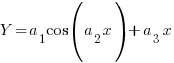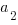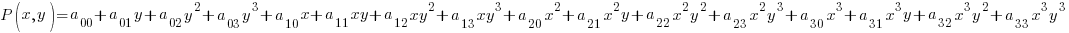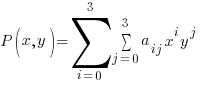# Curve Fitting via SVD

March 7, 2011

I used this method to delete a gradient effect from uneven lighting of some of my book scans.

Here is a simple method for curve fitting various polynomials. This works for non linear equations as long as the actual coeficients are linear.

For example you can NOT fit the following type of function:Note that the coeficientsis an argument to a nonlinear function. related link

The specific one done here is a bicubic polynomial expressed by the following.

We are going to fit the following curve:Or more compactly:``` import cern.colt.matrix.linalg.*;
import cern.colt.matrix.*;
import cern.colt.matrix.impl.*;

import edu.umbc.cs.maple.utils.*;

public DoubleMatrix2D calcFit(ArrayList<Point> measures)
{
int cols = 16; // number of coeficients
int rows = measures.size();  // size of your dataset

DenseDoubleMatrix2D M = new DenseDoubleMatrix2D(rows,cols);
DenseDoubleMatrix2D P = new DenseDoubleMatrix2D(rows,1);

for (int k=0;k<measures.size();k++)
{
Point measure = measures.get(k);  // measures from your dataset
P.set(k,0,measure.getValue());    // Point contains values P , x and y

double x = measure.getX();
double y = measure.getY();

// populate the matrix with the appropriate polynomial values
// from the bicubic above
int col=0;
for (int i=0;i<4;i++)
for (int j=0;j<4;j++)
{
double cal = pow(x,i)*pow(y,j);
M.set(k,col++,cal);
}
}

// solve SVD
SingularValueDecomposition svd = new SingularValueDecomposition(M);
DoubleMatrix2D U = svd.getU();
DoubleMatrix2D V = svd.getV();
DoubleMatrix2D S = svd.getS();

// S is the diagonal matrix
// Invert by replacing its diagonal elements by their reciprocals
for (int i=0;i<16;i++)
{
double val = 1.0/S.get(i,i);

if (S.get(i,i) == 0)  // avoid numeric underflow
val = 0.0;

S.set(i,i, val);
}

Algebra alg = new Algebra();

// X pseudoinverse
DoubleMatrix2D VS = alg.mult(V,S);

DoubleMatrix2D Minv = alg.mult(VS,alg.transpose(U));

// matrix has coeficients
DoubleMatrix2D C = alg.mult(Minv, P);

// If you would like the closeness of the fit use the following

DoubleMatrix2D eP = alg.mult(M,C); // estimated values

ColtUtils utils = new ColtUtils();
DoubleMatrix2D dP = utils.minus(P,eP); // difference between estimated and observed values
double sum = utils.dotproduct(utils.getcol(dP,0),utils.getcol(dP,0));

System.out.println("sum = " + sum);  // how close was our fit?

return(C);
}```

In practice you may need to normalize your measurements if they are large to avoid numeric under and/or over flow.

Tags: ,